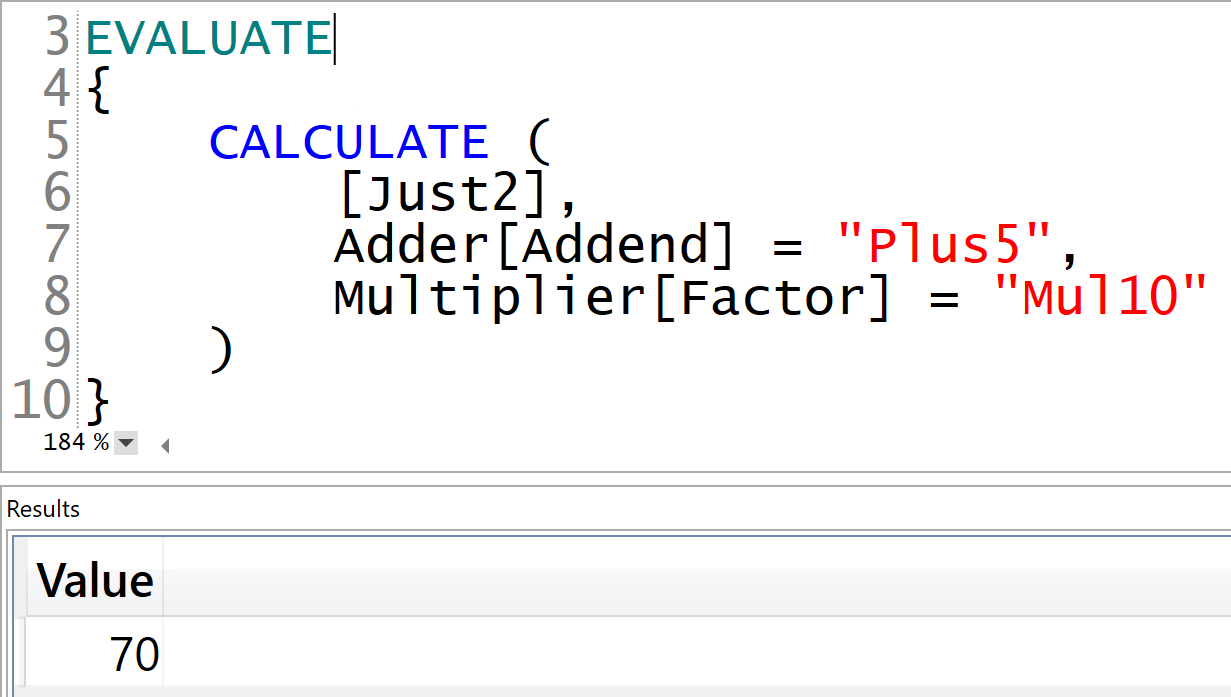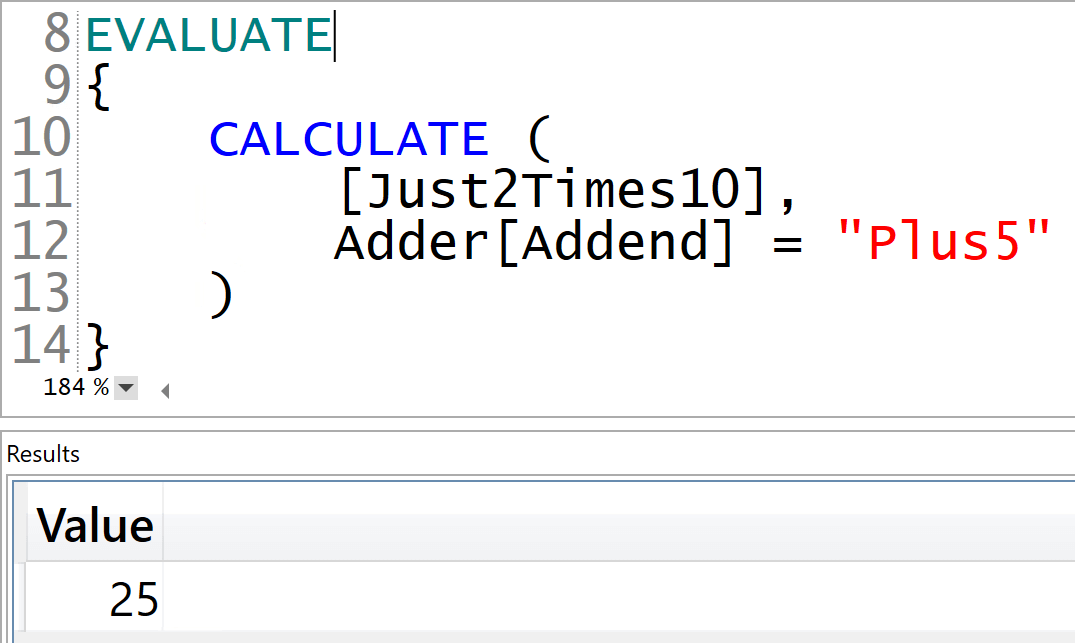When there are multiple calculation groups in a model, we must define their precedence. This ensures we get the expected result when applying different calculation groups to a measure. More details are available in the Understanding Calculation Group Precedence article.

Even in a well-designed model, there are scenarios where a poor combination of calculation groups precedence, CALCULATE, and context transition in a DAX formula can translate into the results being inaccurate. This article shows how much care needs be taken to work with calculation groups.

You create a model with two calculation groups: Adder and Multiplier. The purpose of Adder is to add a number to the selected measure, whereas Multiplier multiplies the selected measure by a factor. Each calculation group contains a single calculation item:

```--
-- Calculation Item: Plus5
--
SELECTEDMEASURE () + 5

--
-- Calculation Group: Multiplier
-- Calculation Item: Mul10
--
SELECTEDMEASURE () * 10
```

Following mathematics rules, we want to apply the multiplication before the addition. Therefore, the Multiplier calculation group has a higher precedence of 200, whereas Adder has a lower precedence of 100. We also define a measure to complete the data model to test in this article: the Just2 measure simply returns the 2 value:

```Just2 := 2
```

The article requires full knowledge of the precedence in calculation groups and the application of calculation items. Before covering the main topic of this article, we warm-up your brain and refresh your knowledge of calculation groups with four small puzzles, each one with a piece of DAX code and the result. Your job is to explain why DAX computed that result. Try to explain the result before reading further. The explanation is right after each figure, and these puzzles are super helpful to train your brain on how to think about calculation groups.

Let us start with the first test: why is the result 2, as if the calculation items were not being applied?The answer is that a calculation item is only applied to a measure reference. If there are no measures in the code, the application item has nowhere to be applied. Because the first argument of CALCULATE is just a constant value, the calculation items are not applied at all. No measure, no application.

Warming up? Good. Now it is time for the second puzzle: why is the result 70 and not 25?The precedence of calculation groups defines the order of application of calculation items. The application is not an evaluation. Multiplier is applied first, and then Adder is applied later. Therefore, the code follows these two transformations:

```--
--    This the initial expression
--
CALCULATE (
[Just2],
Multiplier[Factor] = "Mul10"
)
--
--    The first calculation item being applied is Multiplier
--
CALCULATE (
[Just2] * 10,
)
--
--    The second calculation item being applied is Adder
--
( [Just2] + 5 ) * 10
```

The order of the calculation items in CALCULATE does not affect the result. Calculation items are only applied following the precedence of their corresponding calculation groups.

Now, the third difficult question. Can we force the order of evaluation of calculation items by using CALCULATE? Why does not DAX follow the order we try to force by using nested CALCULATE functions?The answer requires a bit more attention here, although the explanation is the same as the previous ones. A calculation item is applied on a measure reference. The outer calculation item from Adder does not have any effect on the inner CALCULATE, because it is applied to the Just2 measure only inside the inner CALCULATE. Therefore, this code is identical to the previous puzzle. Nesting CALCULATE functions is not a way to change the order of application of calculation items, at least not in this example. Things can be much more complicated if we have multiple measure references instead of a simple CALCULATE. Nevertheless, the goal of this article is not to write more complex code. Instead, we want to show how to correctly understand the result of an expression when calculation groups are involved.

Let us complicate the scenario in the fourth puzzle. We define a new Just2Times10 measure:

```Just2Times10 :=
CALCULATE (
[Just2],
Multiplier[Factor] = "Mul10"
)
```

Then, we run the following query: we finally obtain 25 as a result, but why?The answer is still the same: calculation items are applied on a measure reference. In this case, the measure reference is no longer Just2. Because the CALCULATE function evaluates the Just2Times10 measure, the calculation item is applied to the Just2Times10 measure. Therefore, Adder works on Just2Times10 and Multiplier kicks in only later, when Just2Times10 is being evaluated:

```--
--    This the initial expression
--
CALCULATE (
[Just2Times10],
)
--
--    The only calculation item being applied is Adder
--
[Just2Times10] + 5
--
--    Just2Times10 invokes another calculation item
--
CALCULATE (
[Just2],
Multiplier[Factor] = "Mul10"
) + 5
--
--    Only now does Multiplier kick in
--
( [Just2] * 10 ) + 5
```

The code we have seen so far is only a refresher of calculation groups. This warm-up is the longer part of the article. The end goal is to explain that there is a big difference between using a calculation item in CALCULATE and defining a measure that applies the calculation item. When a measure formula applies a calculation item to another measure reference, it overrides the precedence of the calculation groups – whereas when the same CALCULATE applies multiple calculation items, the DAX engine chooses the precedence based on the precedence of the calculation groups involved.

For example, the following query contains two EVALUATE statements. The first EVALUATE groups by Adder[Addend] and evaluates the Just2Times10 measure, whereas the second EVALUATE evaluates the Just2 measure grouped by both Adder[Addend] and Multiplier[Factor]. The results of the two EVALUATE statements are different:The first EVALUATE groups by Adder[Addend].  For this reason, Just2Times10 is included in an implicit CALCULATE function that applies to each row of the result, a different calculation item from the Adder calculation group. The result produced by the Just2Times10 measure reference is 25, as in our last puzzle.

The second EVALUATE groups by both Adder[Addend] and Multiplier[Factor], so there is only one CALCULATE with the two calculation items applied to the Just2 measure. DAX applies the calculation items according to the precedence of the corresponding calculation groups and the result is 70.

At the risk of sounding pedantic, let us repeat the concept once more. If you define a measure that applies a calculation item, you are not doing the same operation as if you add a calculation item to a slicer or rows or columns of a visual. By using a measure, you enforce the application order. In contrast, by using a report filter, you are letting the engine choose the application order based on the precedence setting of the calculation groups. These are not the same thing.

As you have seen, the scenario is rather complex to analyze and understand. Yet, as a DAX expert, you want to handle this complexity successfully. So approach any model you build by making sure that precedence, measures, and calculations are all fine-tuned to provide a smooth user experience. As soon as users decide to create their own measure in a report by using a calculation group with CALCULATE, they have the power to break your well-engineered calculations.

Multiple calculation groups in a model can be extremely complex to manage. Before using them in your models, make sure you take the time to understand how they work in depth, and to understand what the implications of using them can be. Well, do not just take the time. Invest lots of time! The good news is that calculation groups are extremely powerful once you master them. Remember: with great power comes great responsibility!

CALCULATEContext transition

Evaluates an expression in a context modified by filters.

`CALCULATE ( <Expression> [, <Filter> [, <Filter> [, … ] ] ] )`